# R S Aggarwal Solutions for Class 11 Maths Chapter 18- Solution of Triangles

Solution of Triangles discusses the fundamental concept of solutions related to triangles. It is thus necessary to learn the concept and understand its applications with utmost clarity. One of the best resources that can be used to practice the problems related to “Solution of Triangles” is the R S Aggarwal Solutions for Class 11 Maths Chapter 18- Solution of Triangles. These solutions are designed by expert faculties who can articulate concepts in an efficient manner.

Hence, R S Aggarwal Solutions is the guide you should adopt for your studies as the content is presented in a comprehensive format. Furthermore, we ensure that the instructions provided are simple and clear cut.

### Download PDF of R S Aggarwal Solutions for Class 11 Maths Chapter 18 – Solution Of Triangles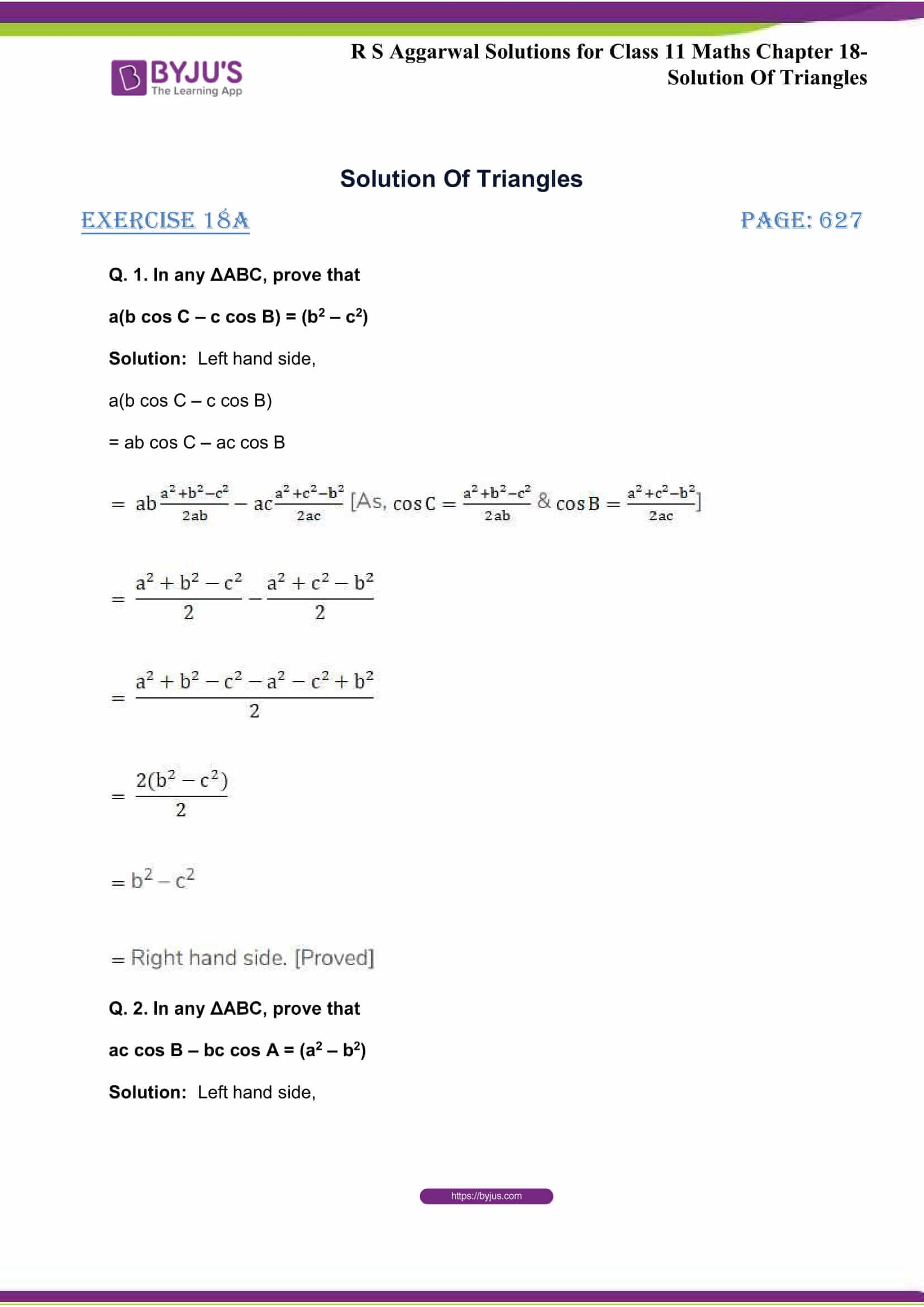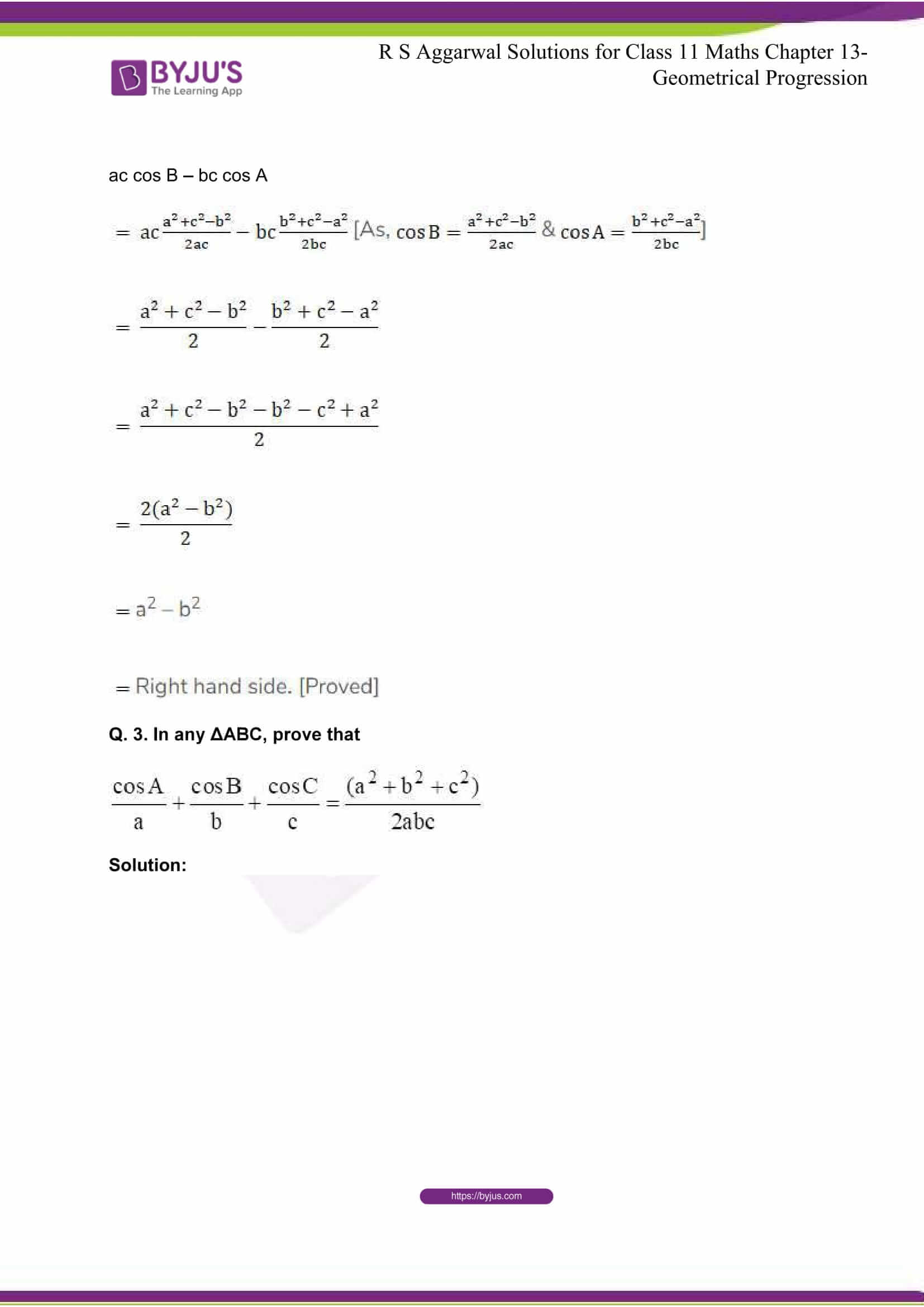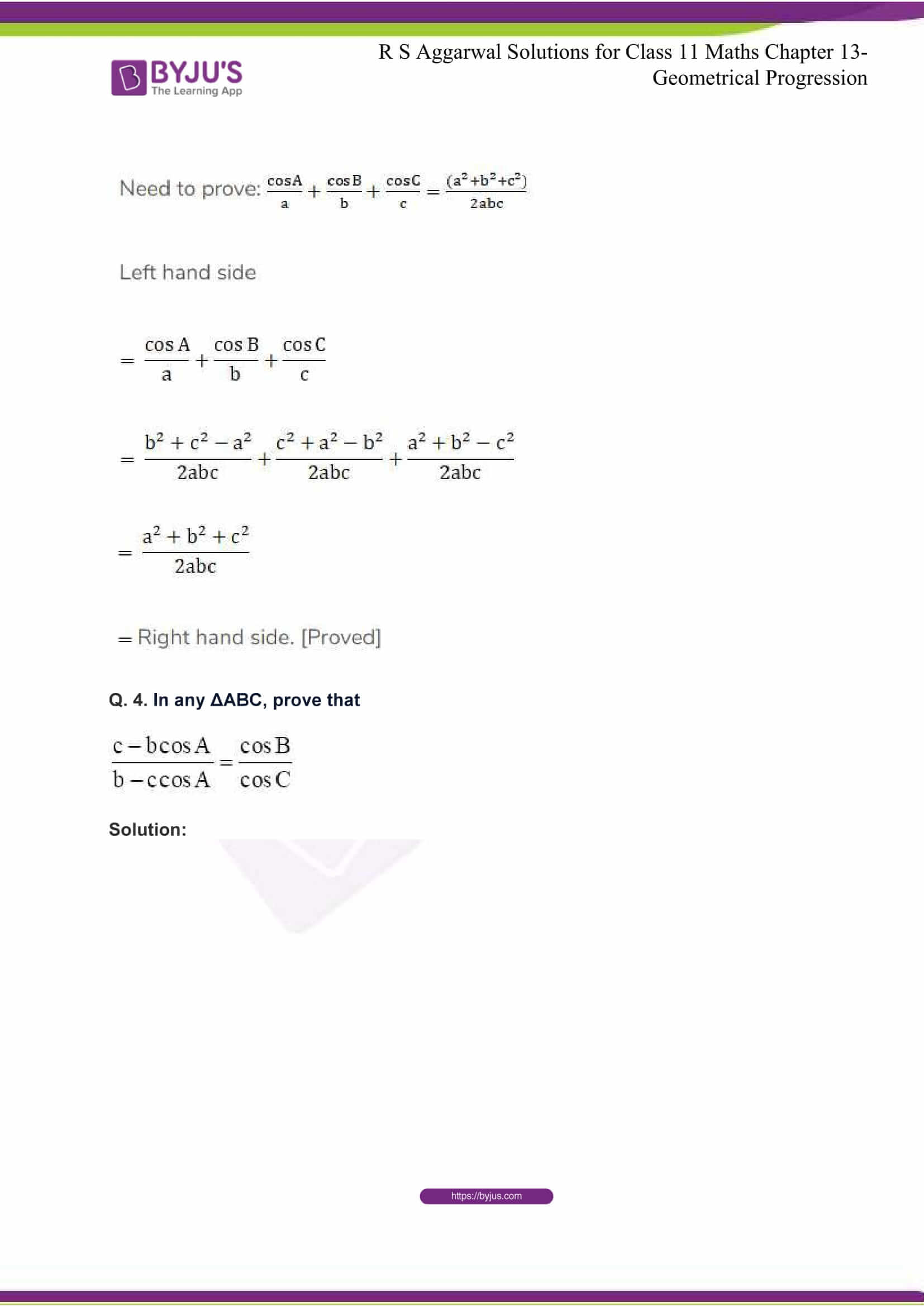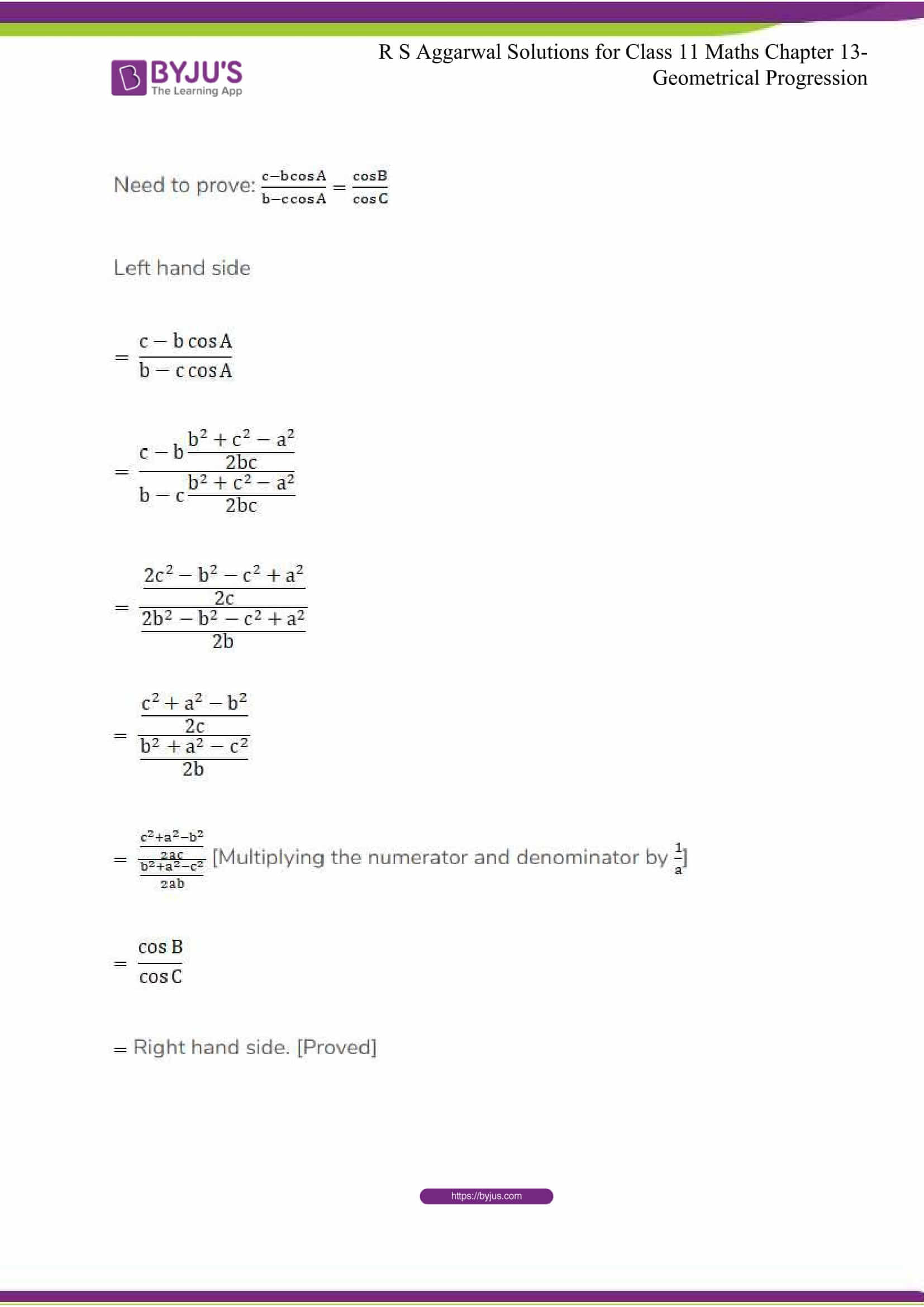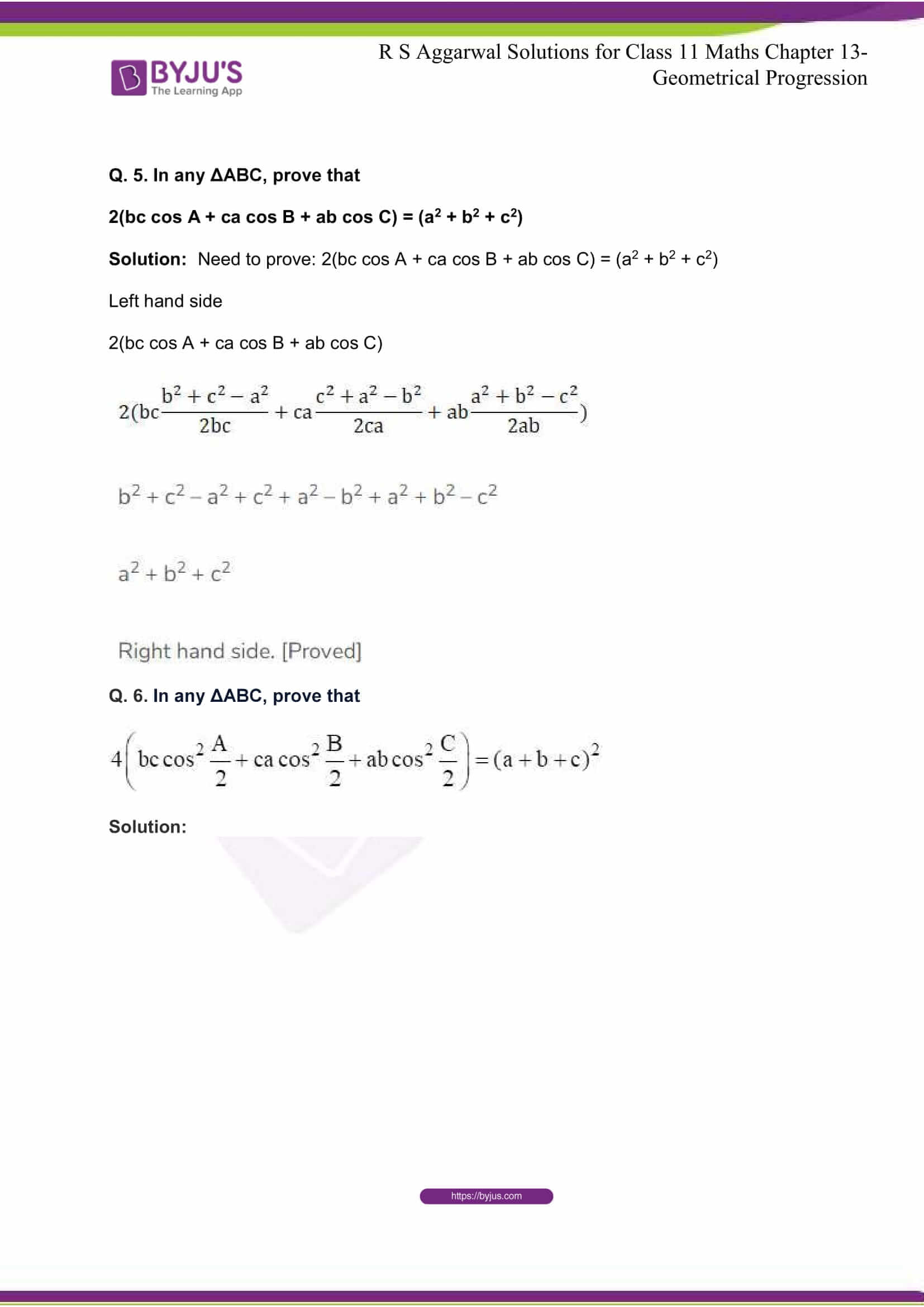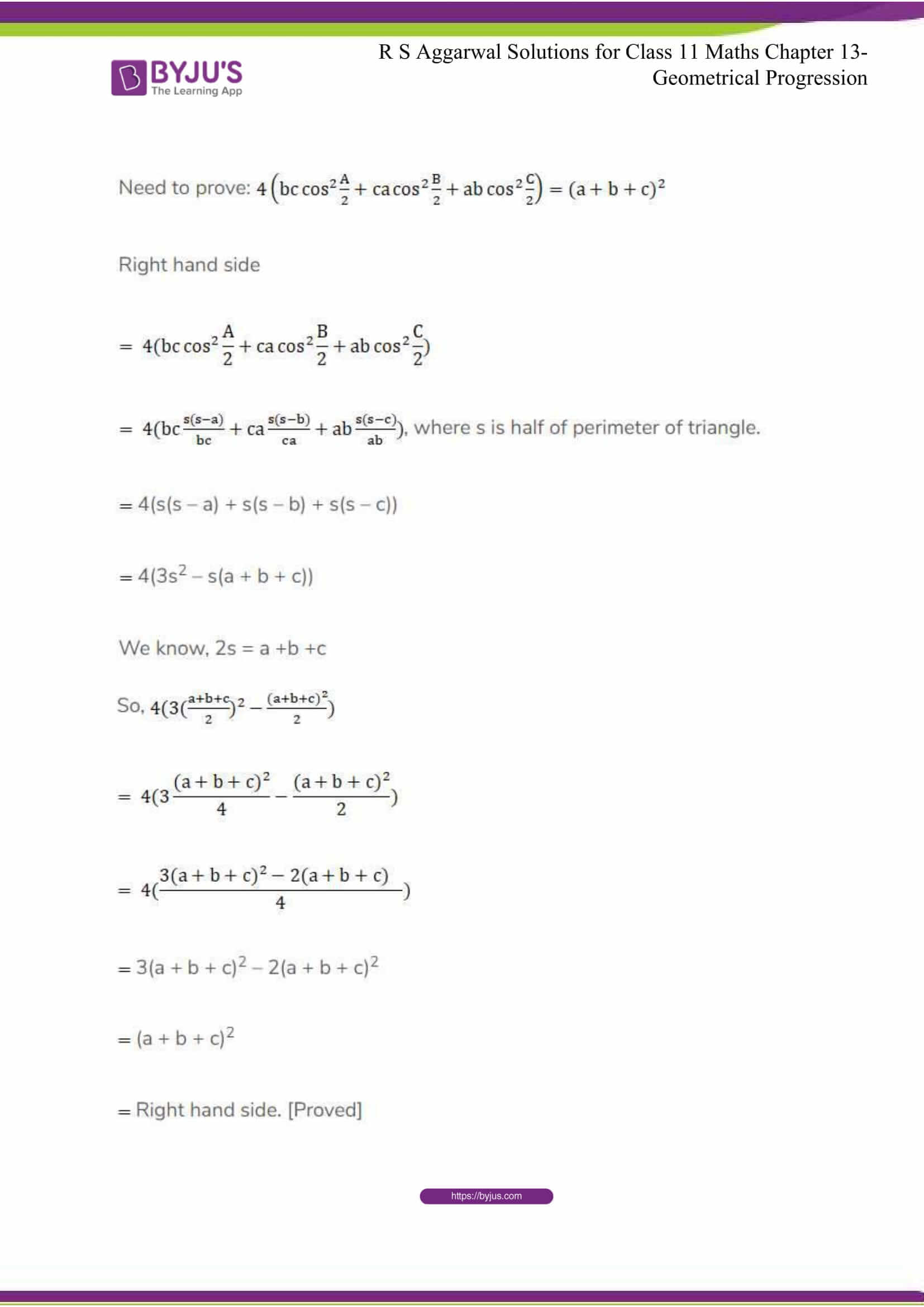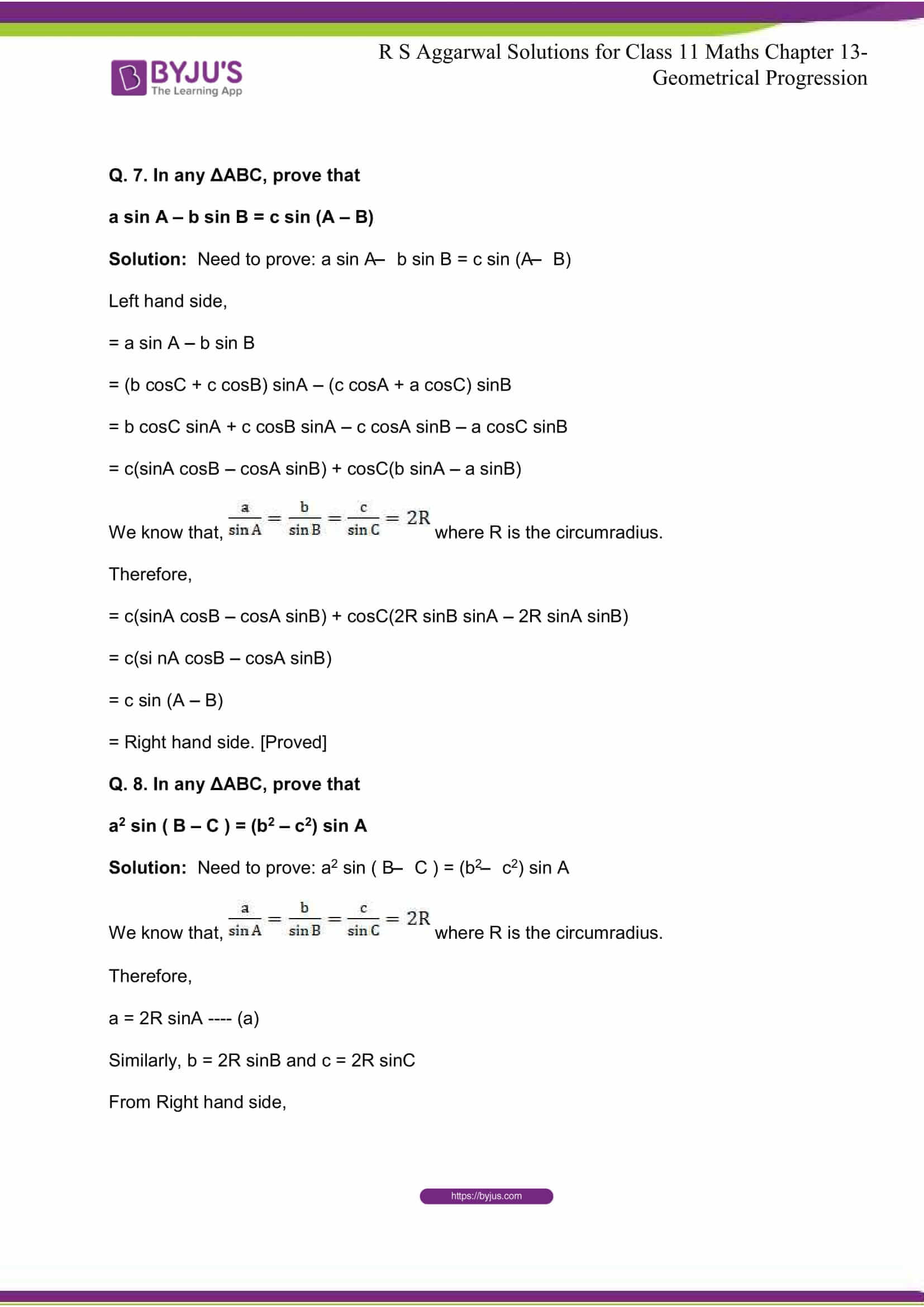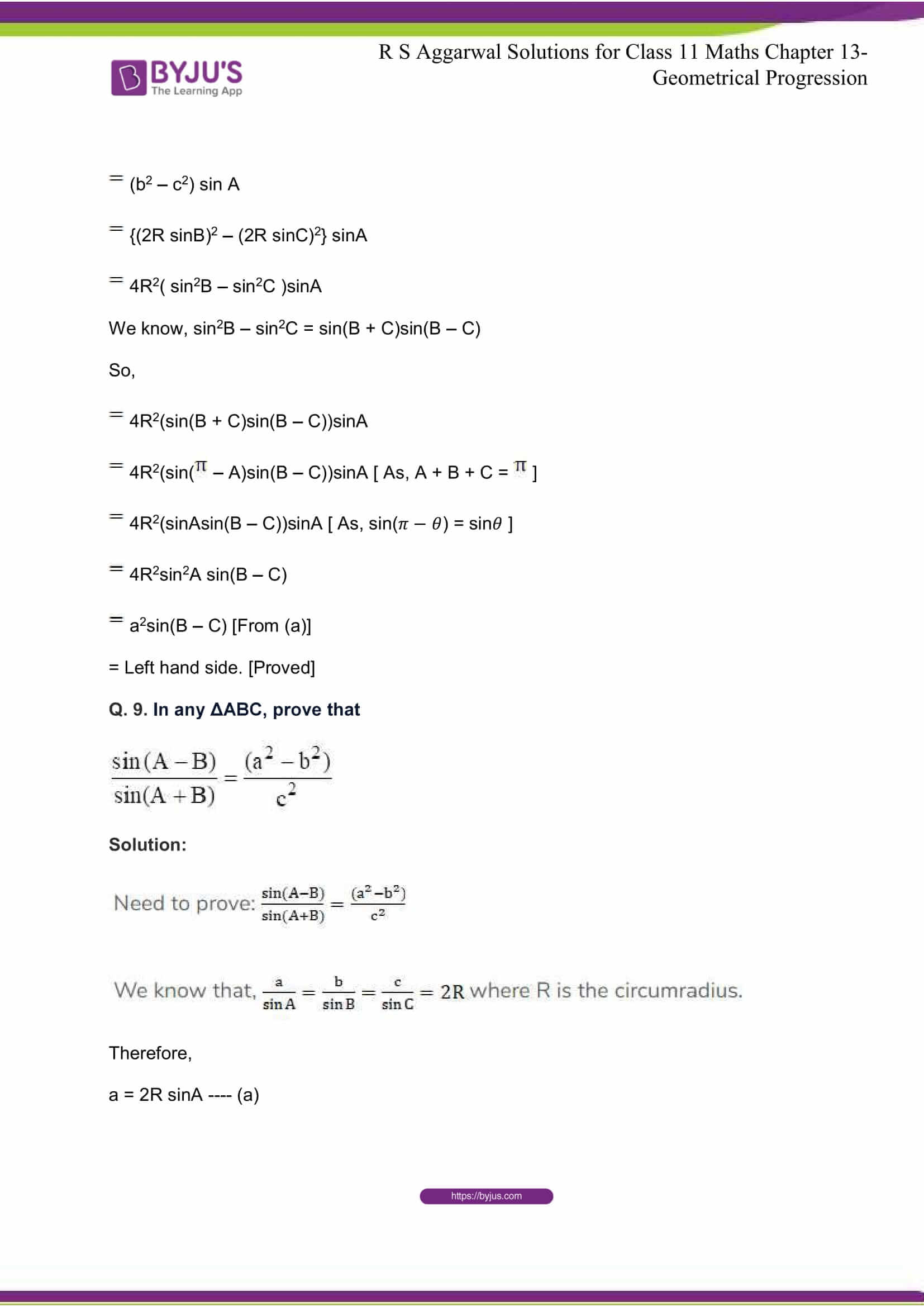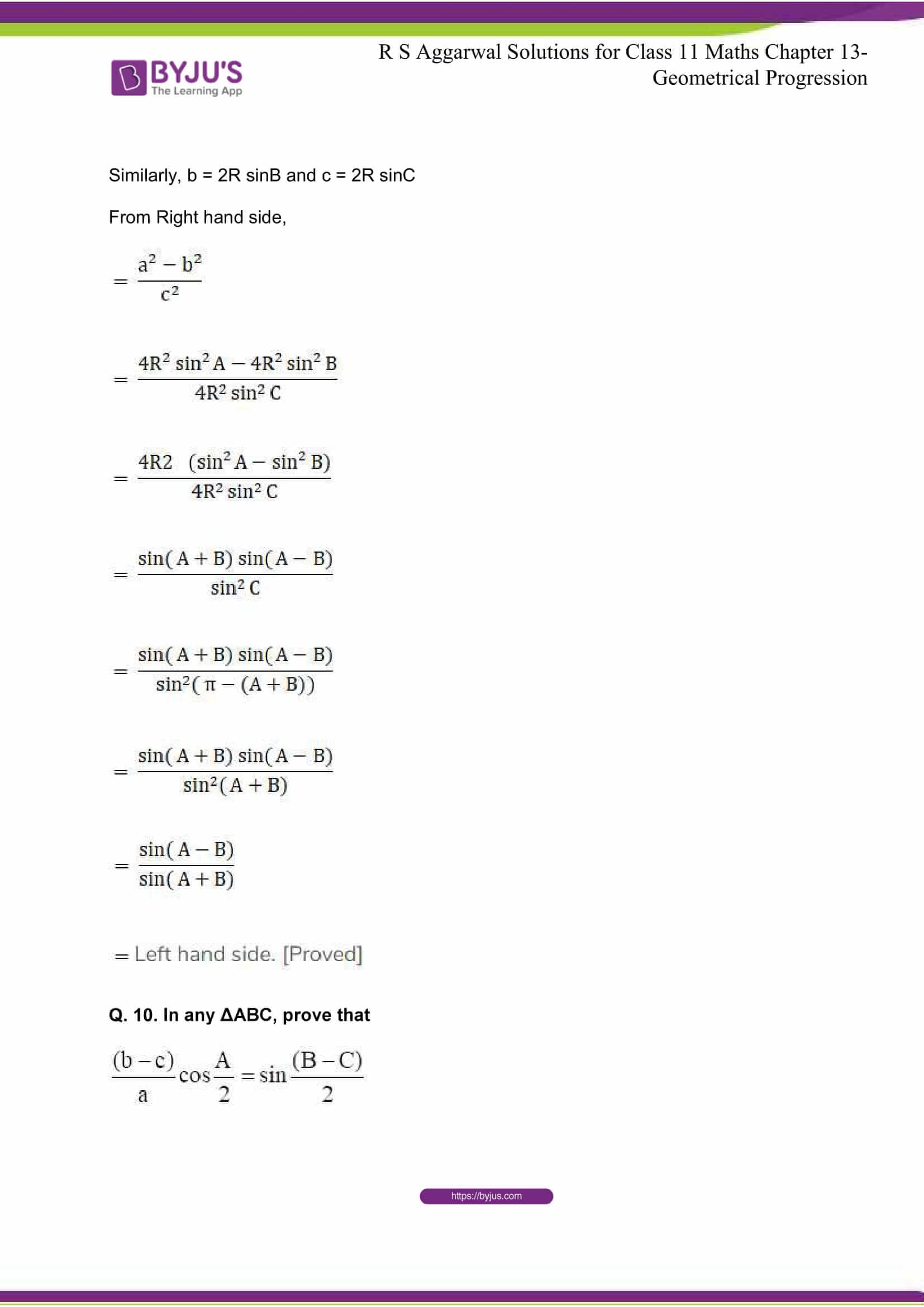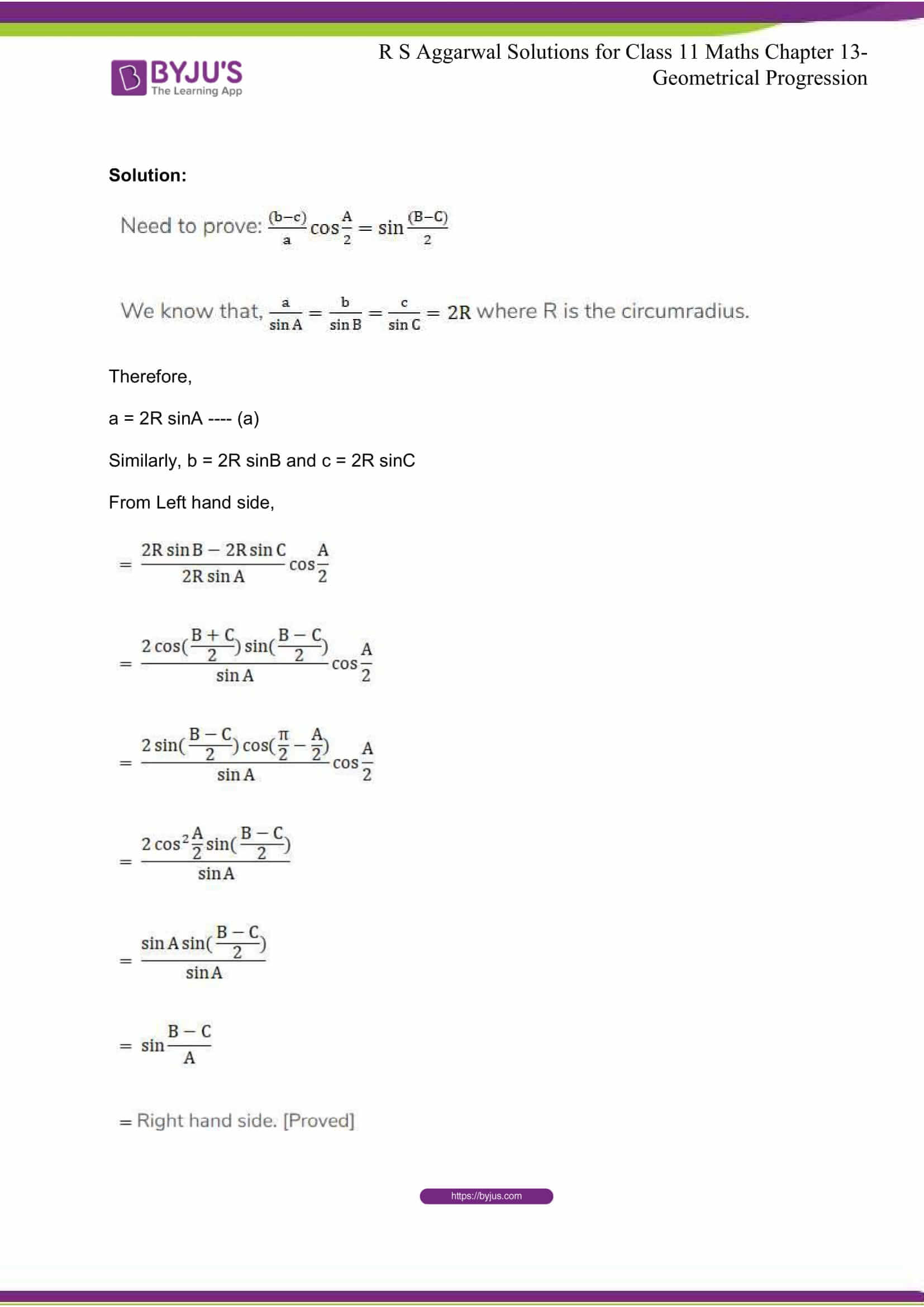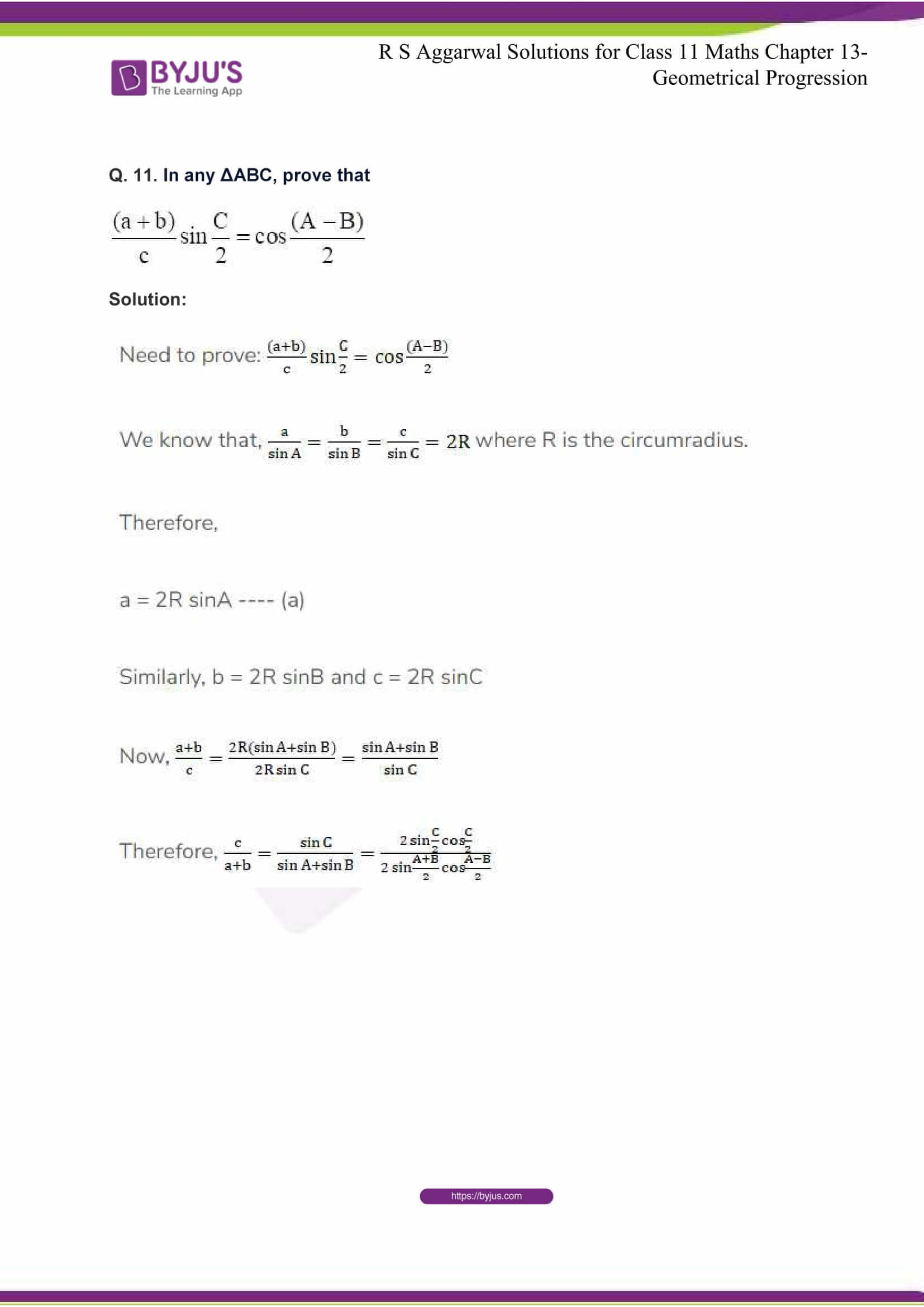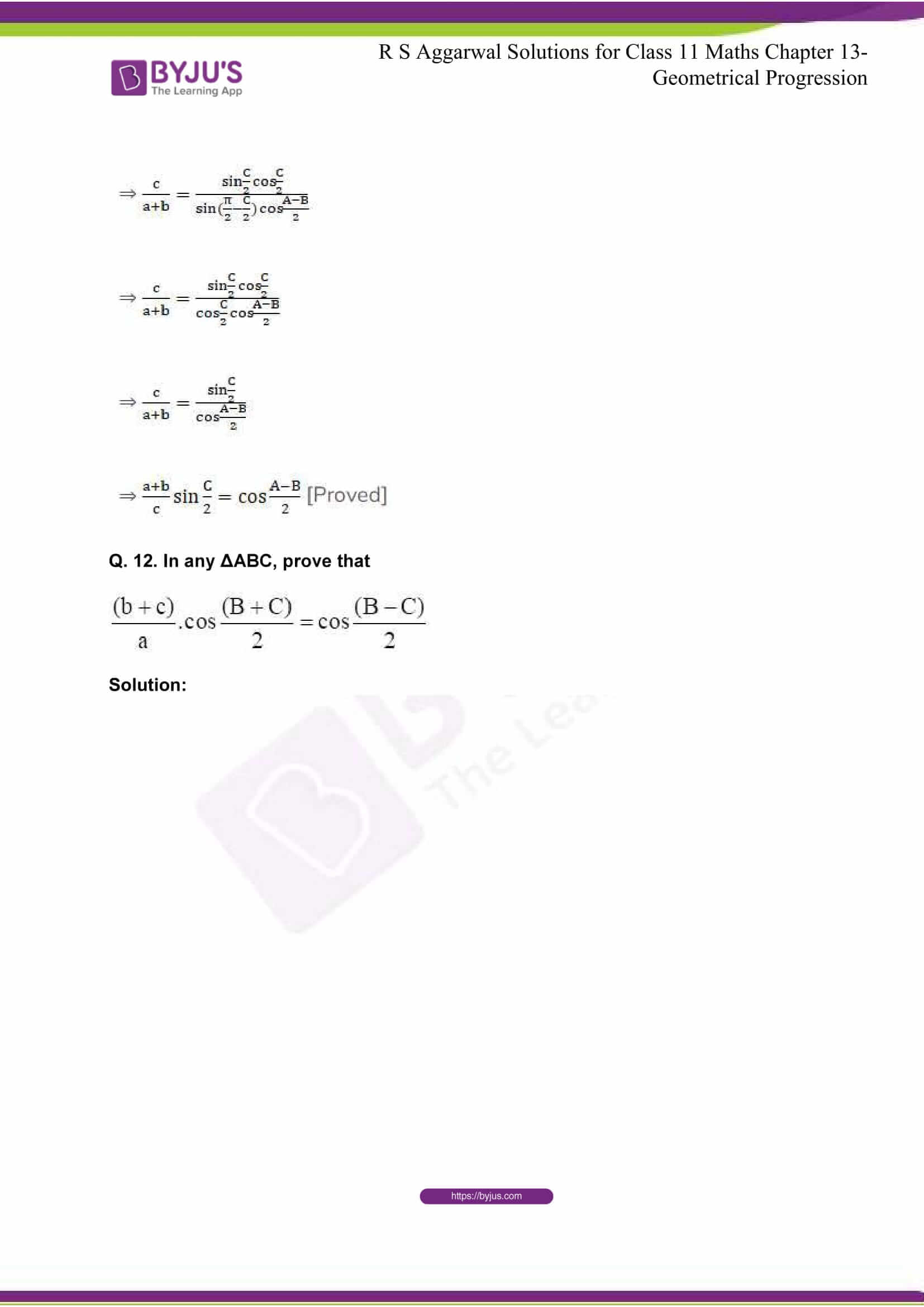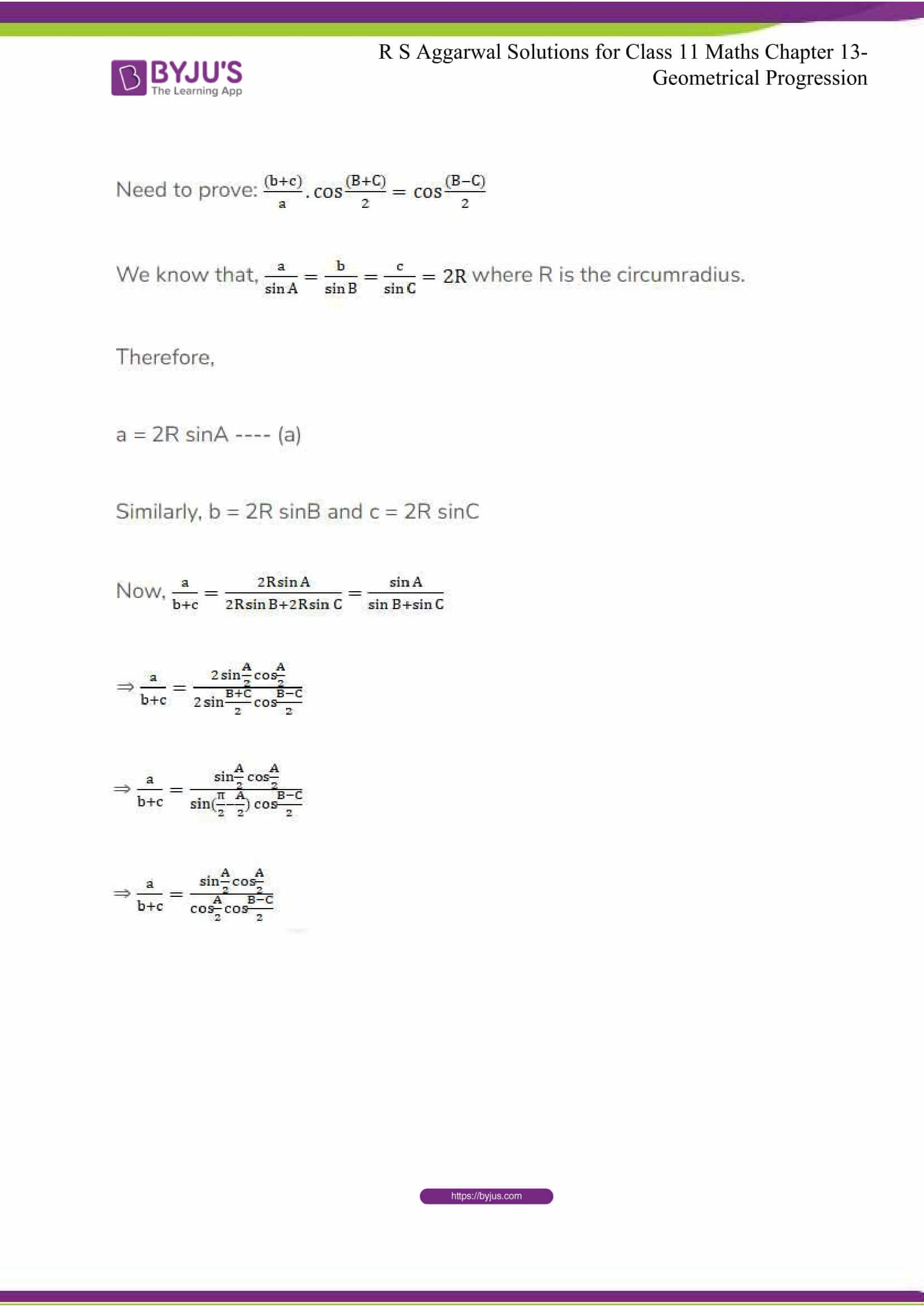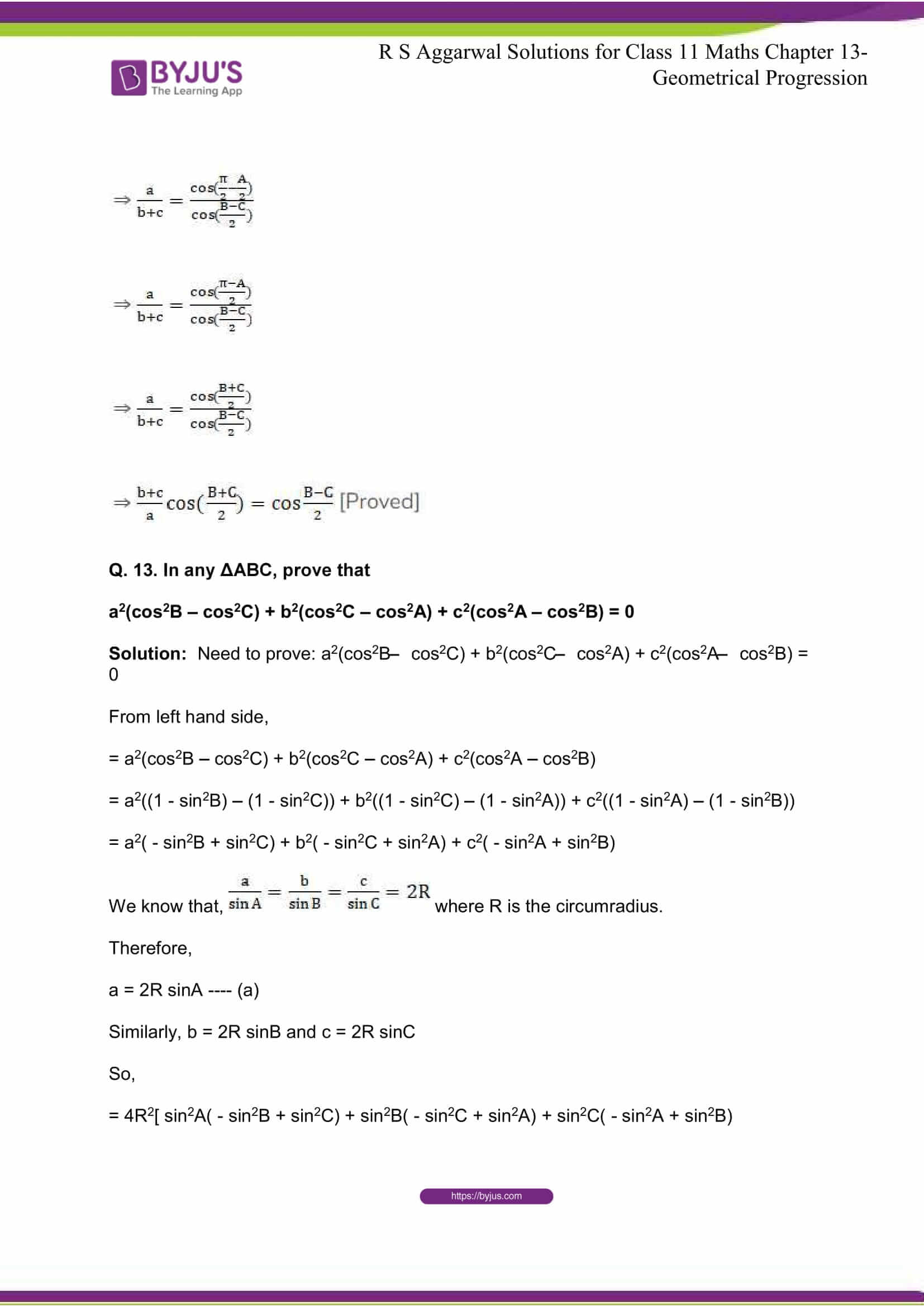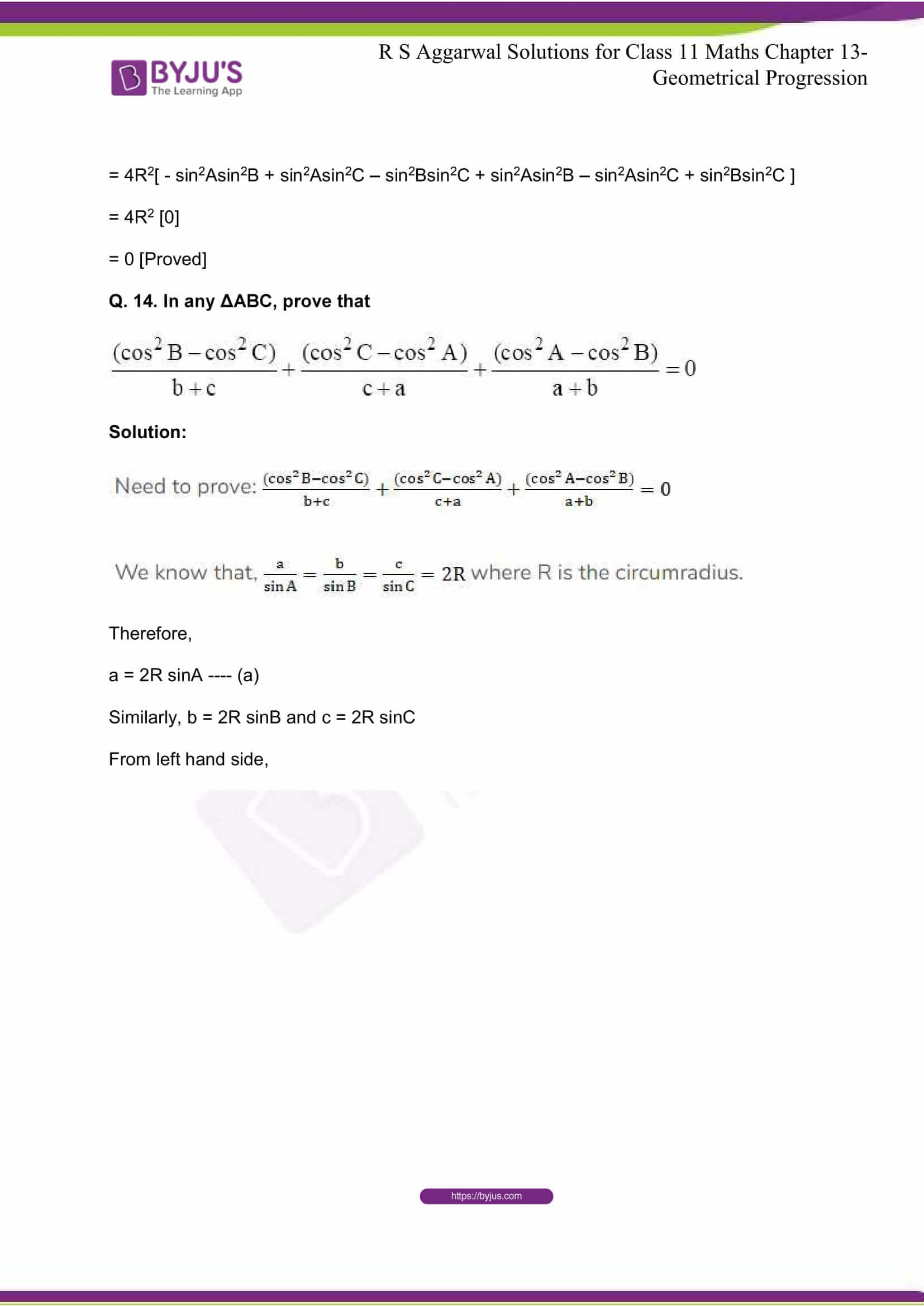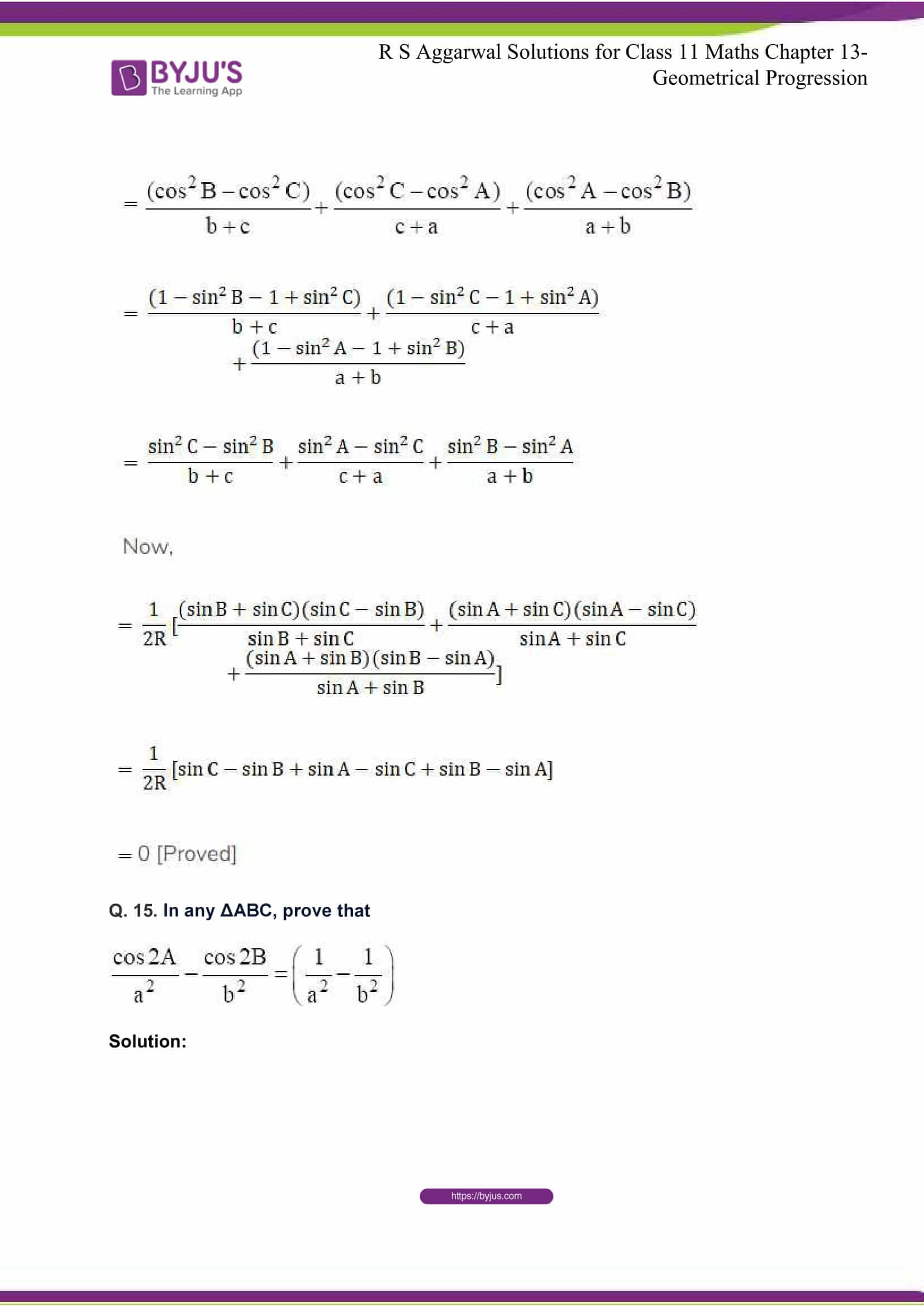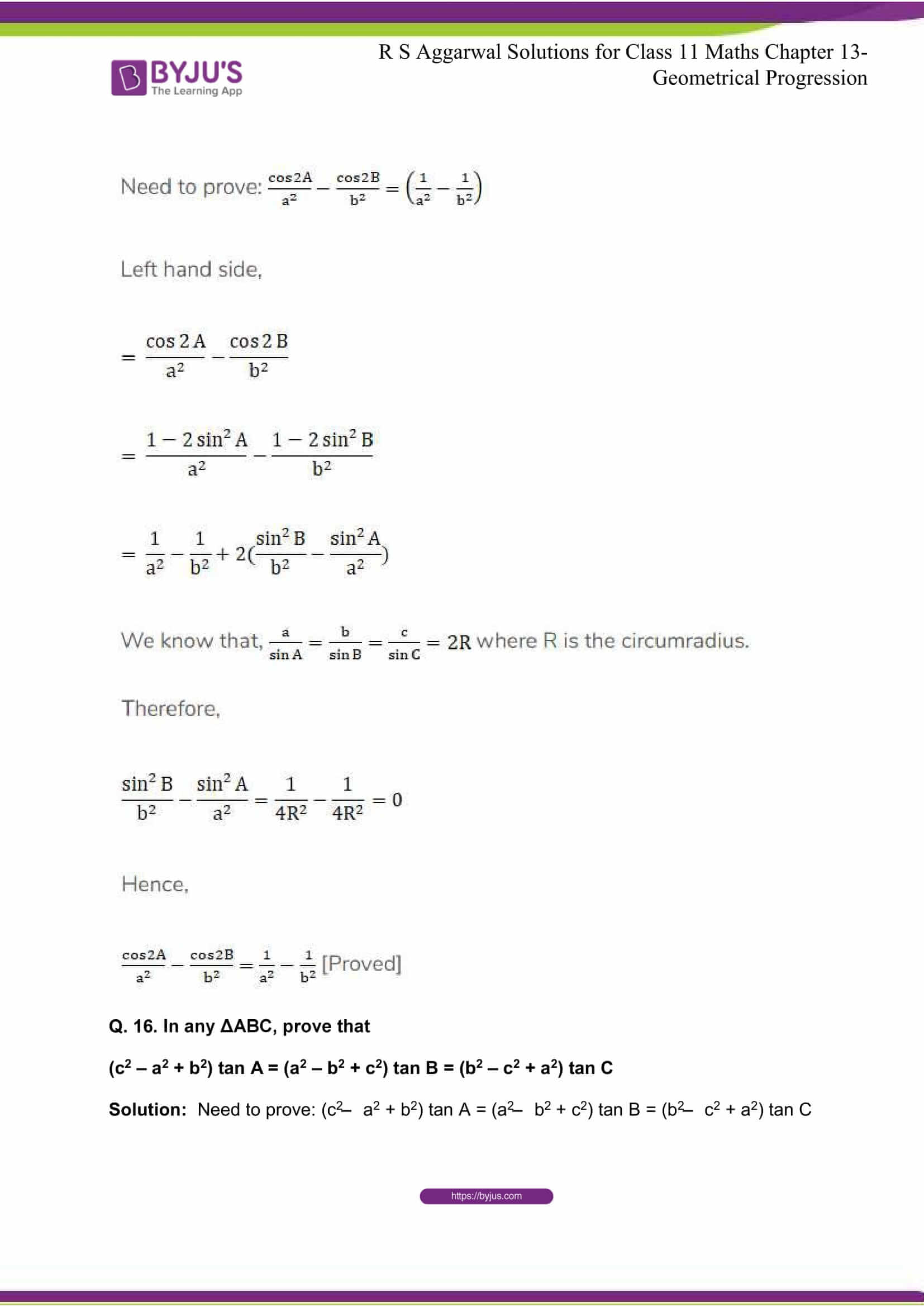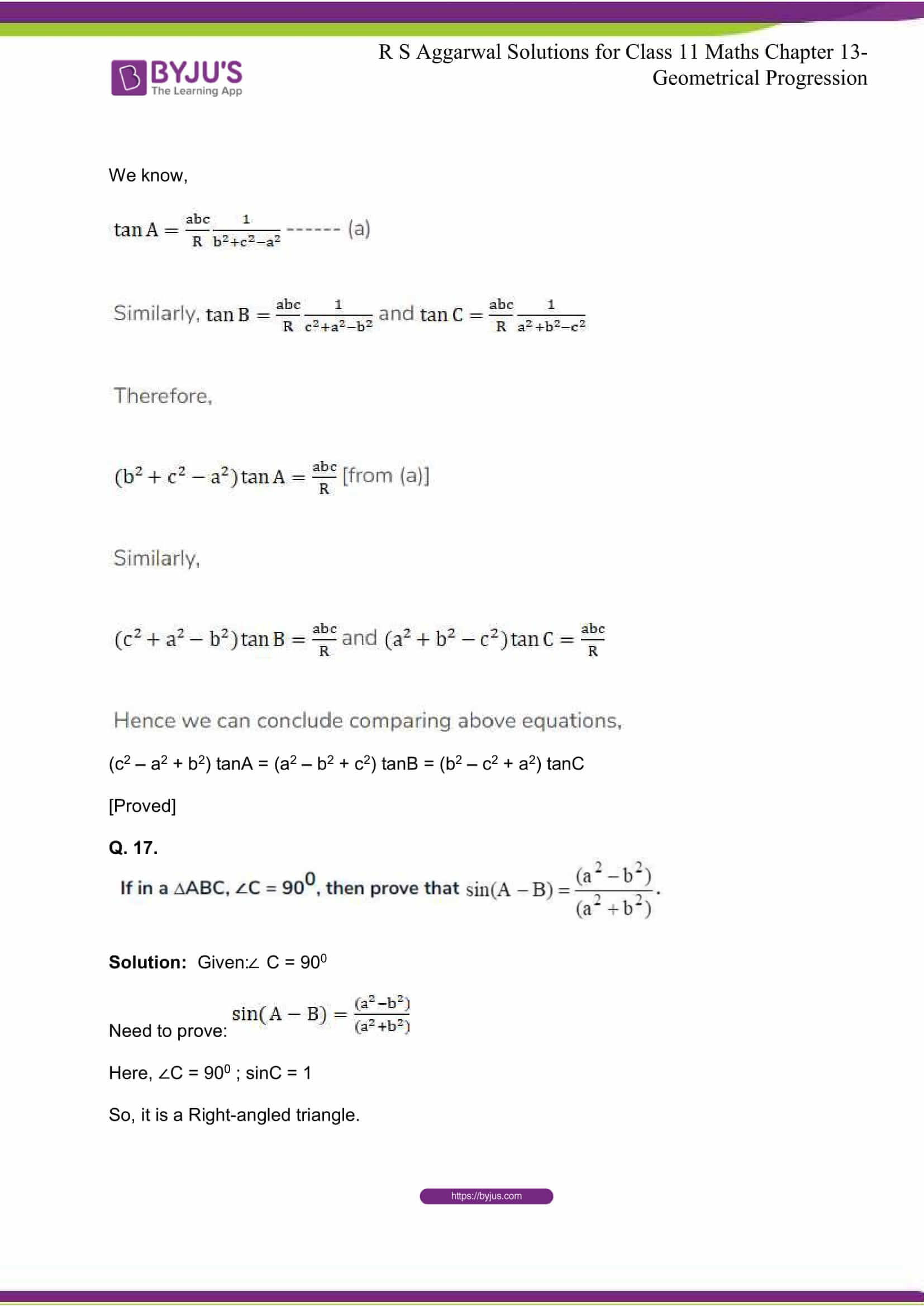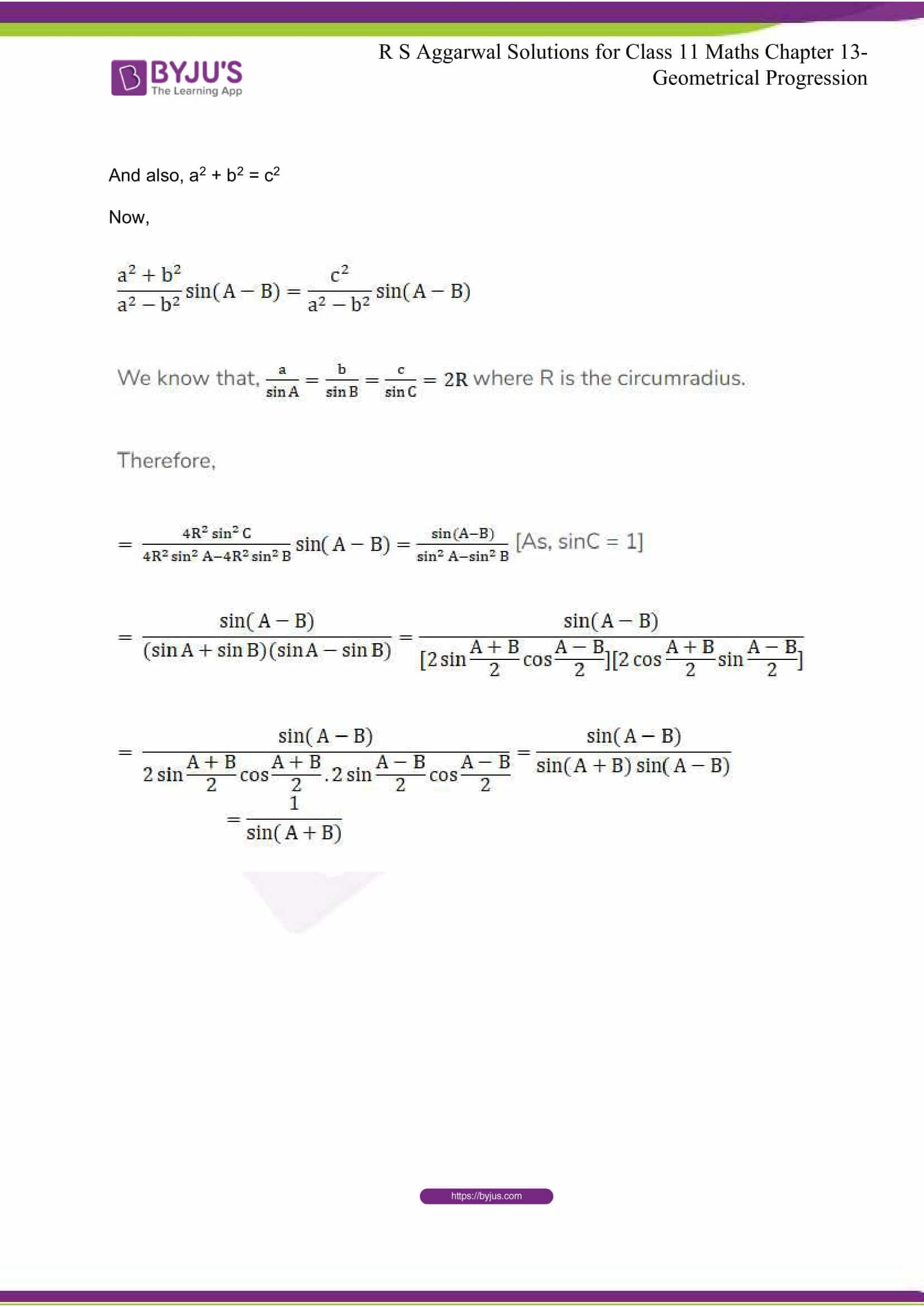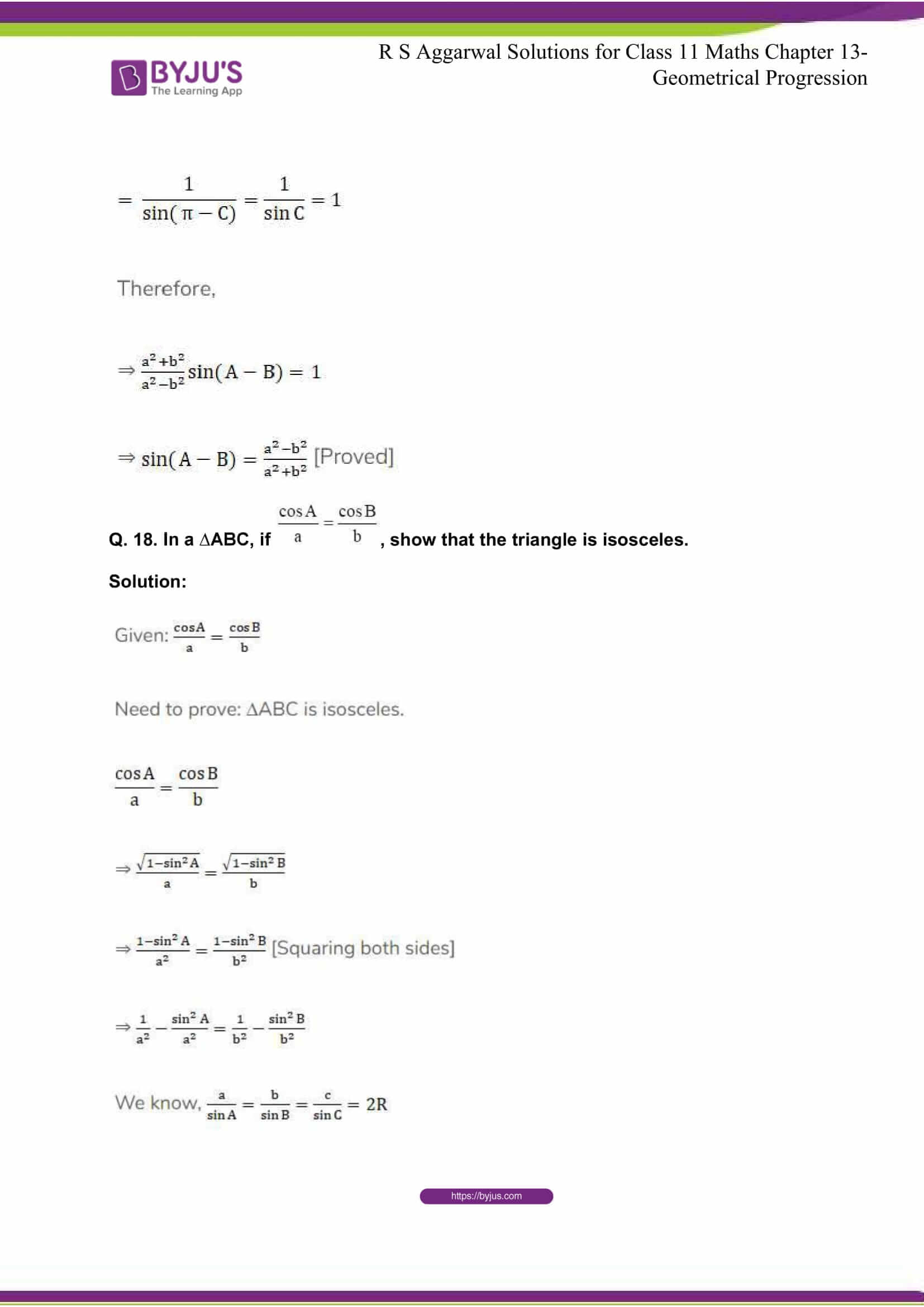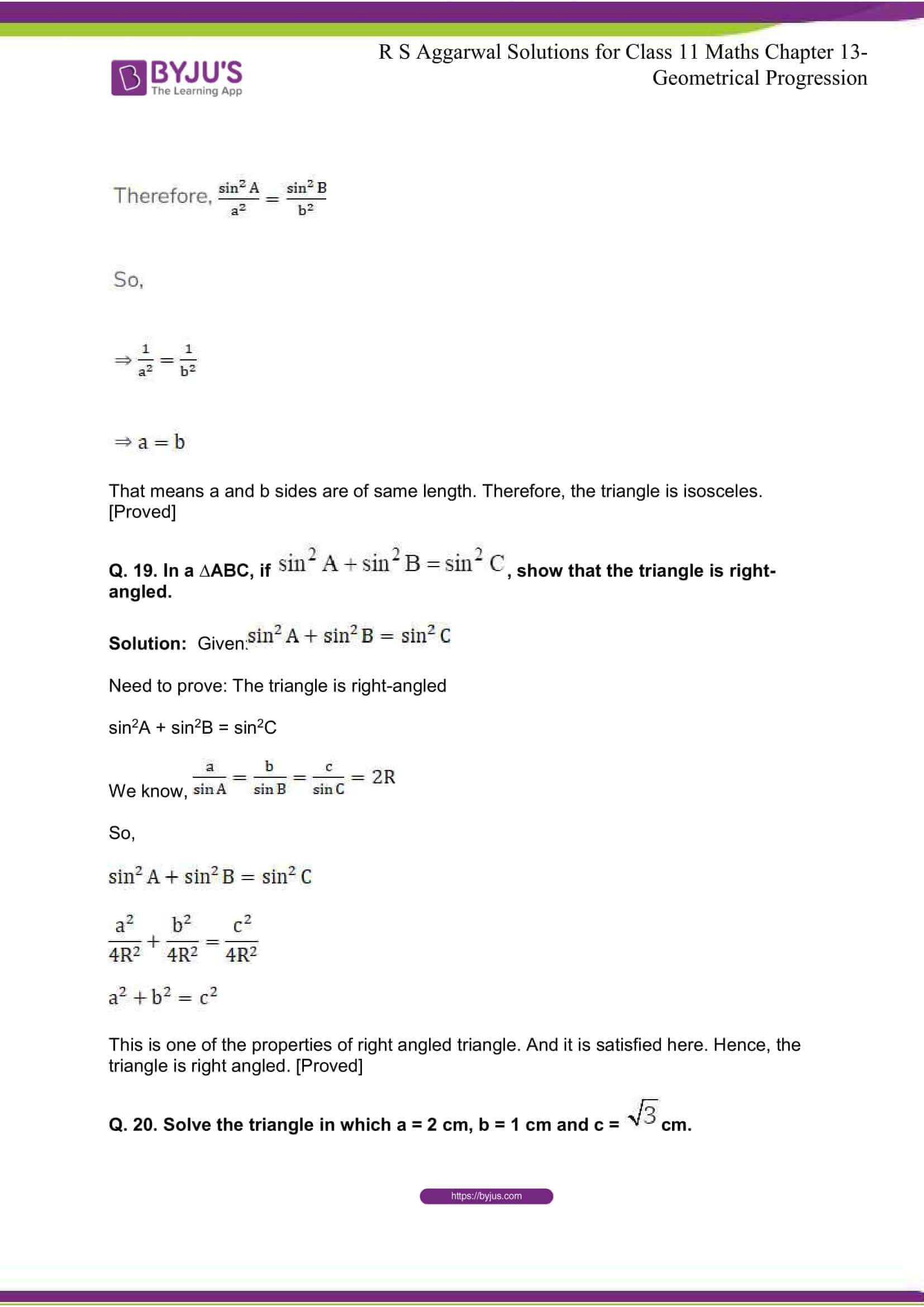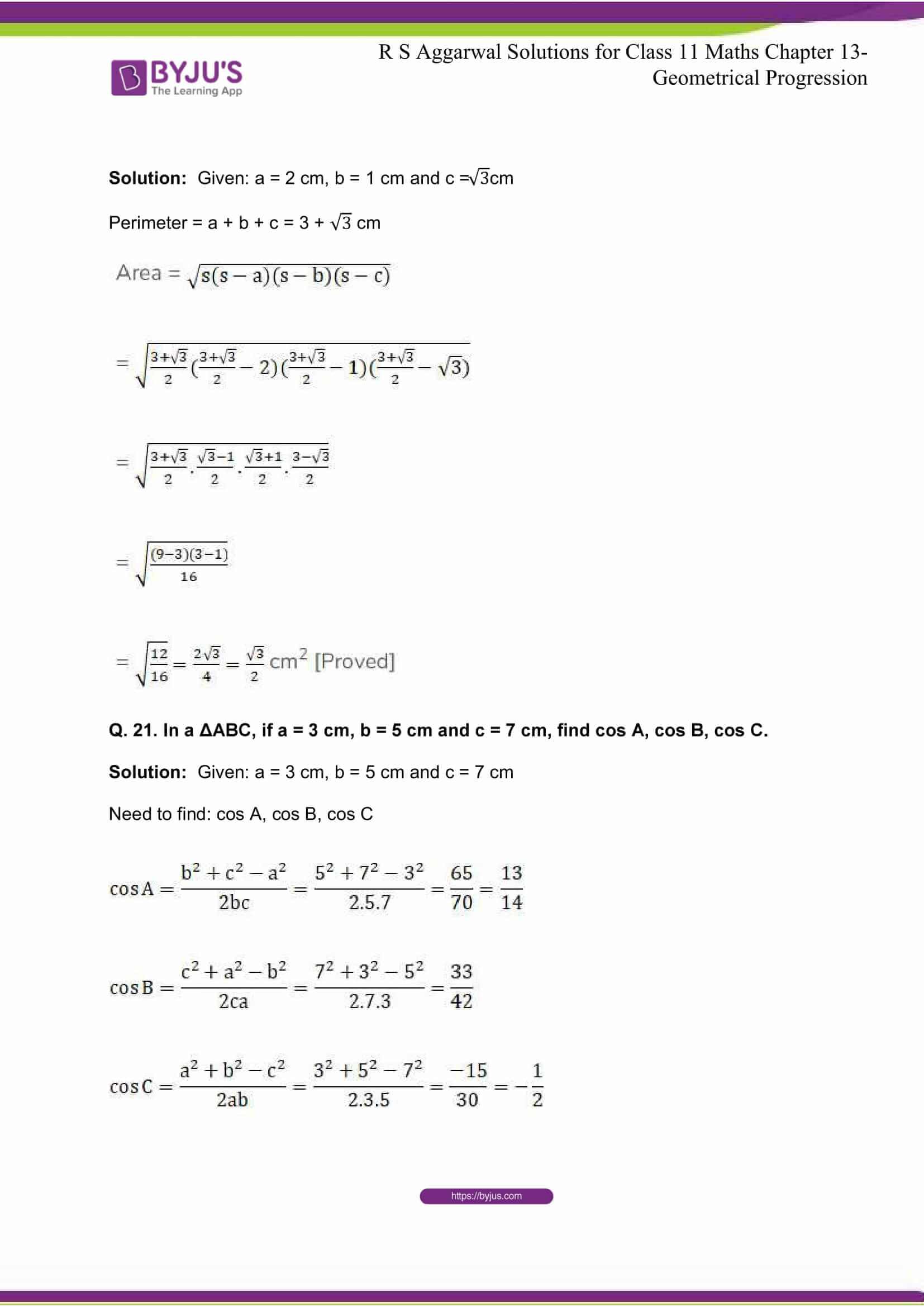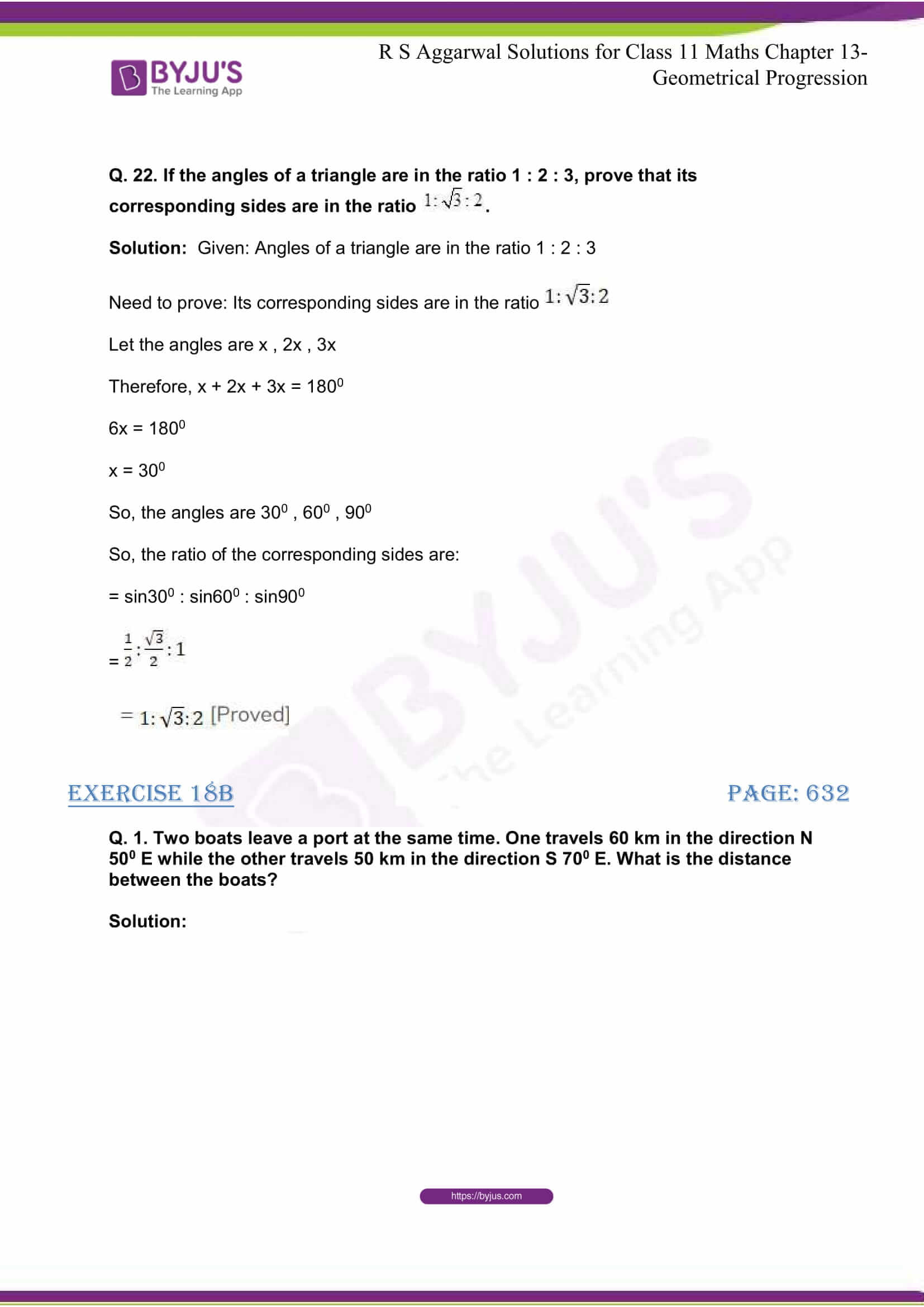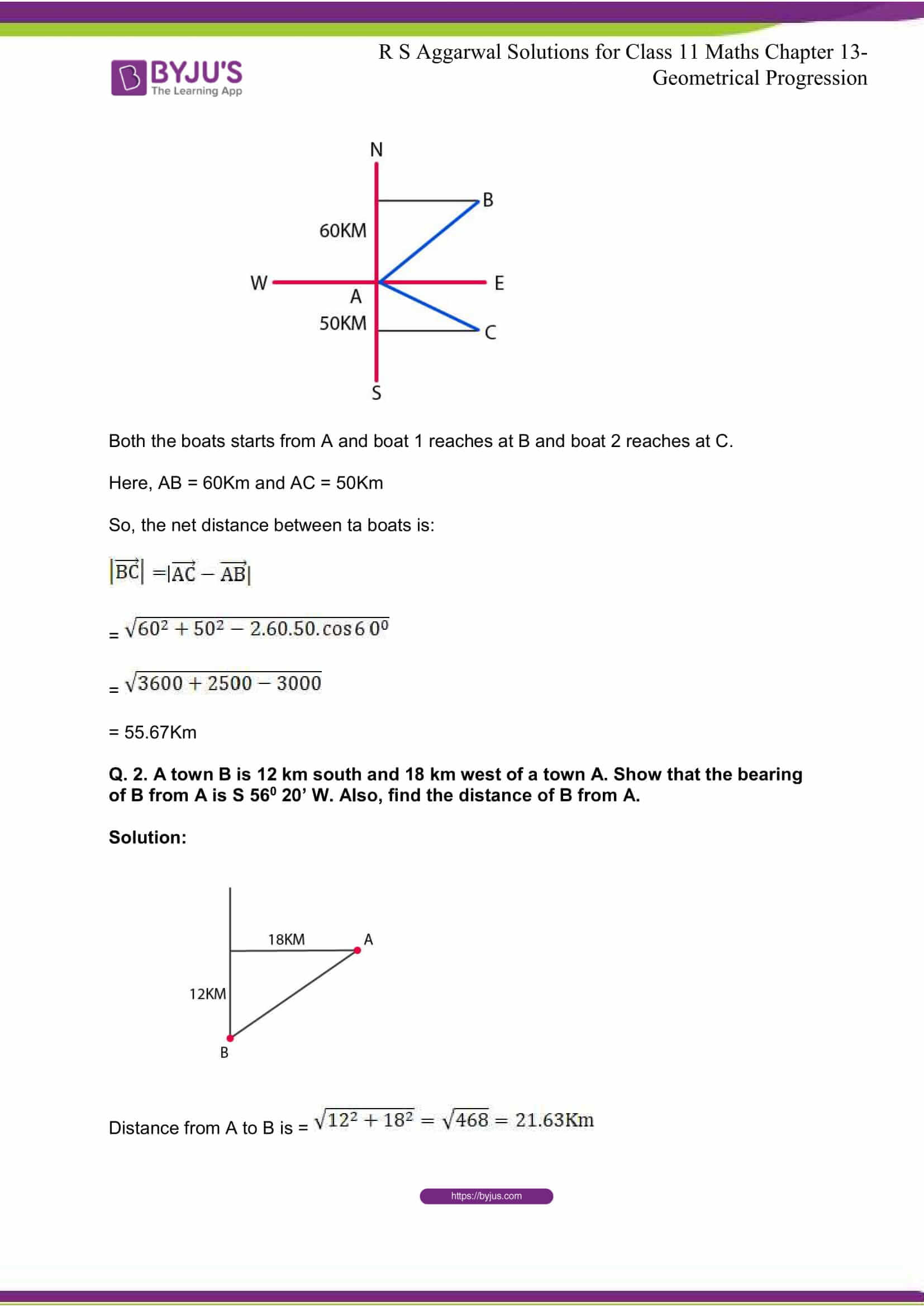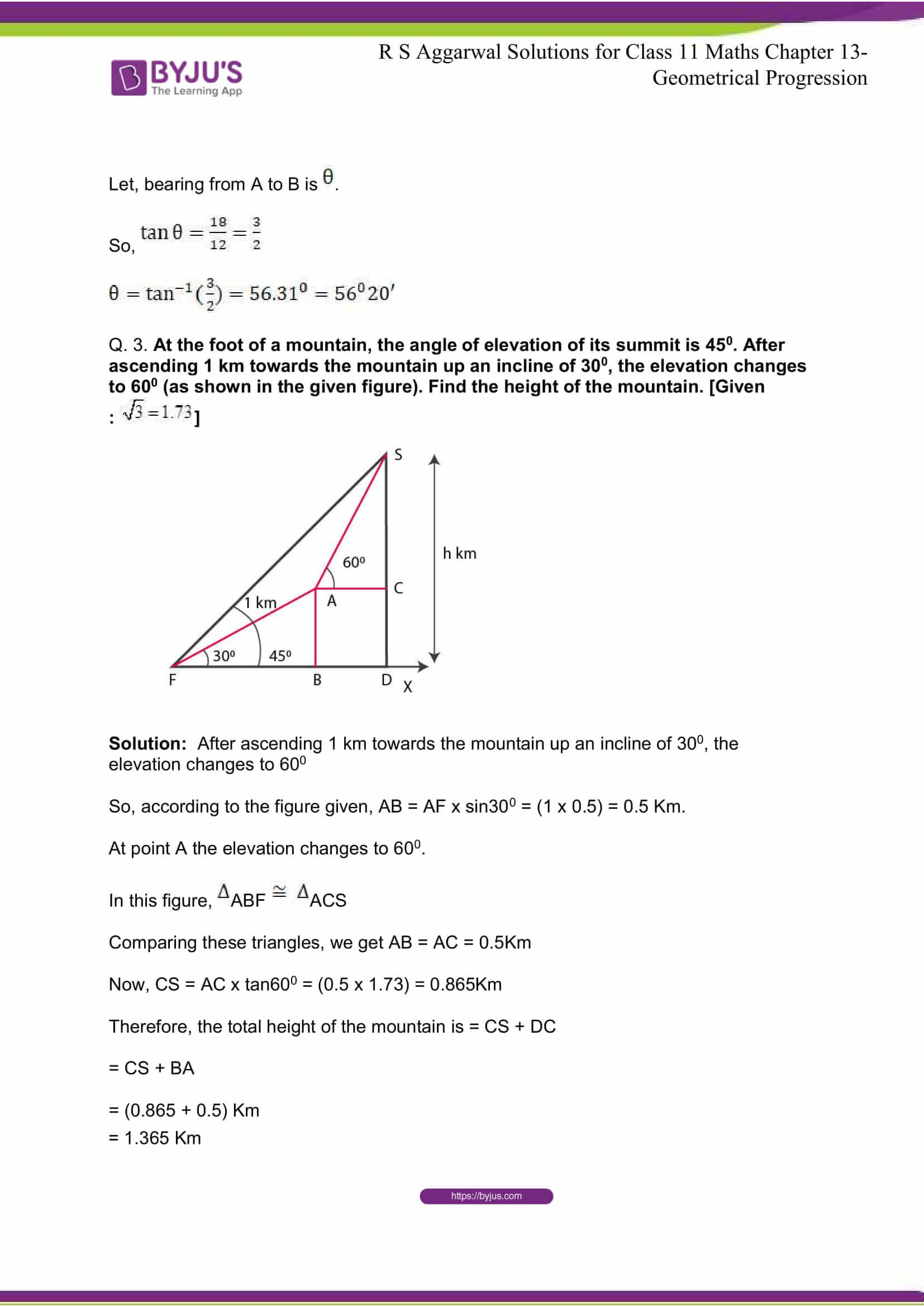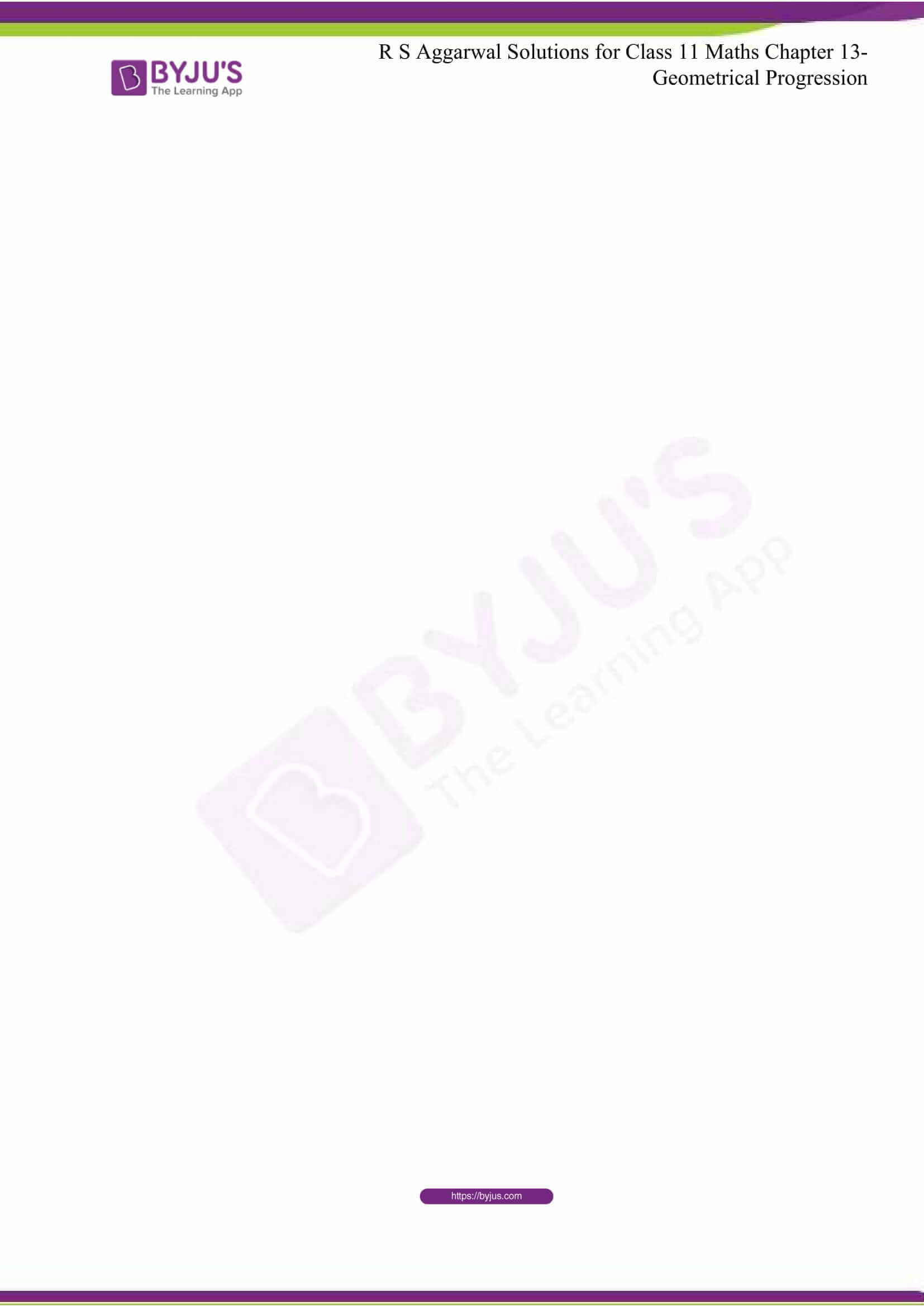## R S Aggarwal Solutions for Class 11 Maths Chapter 18 – Solution Of Triangles

The Chapter 18, Solution Of Triangles, contains 2 exercises and the R S Aggarwal Solutions given here has the answers to all the questions present in these exercises. Let us have a look at some of the topics that are being discussed in this chapter.

18.1 Introduction

18.2 The Oblique Triangle

18.3 Sine Formula

18.4 Cosine Rule

18.5 Napier’s Analogies

18.6 Problems Based on Sine and Cosine Formula

## R S Aggarwal Solutions for Class 11 Maths Chapter 18 – Solution Of Triangles

Solution of triangles is the main trigonometric problem used for finding the characteristics of a triangle when some of angles or lengths of sides of the triangles are known. The triangle can be located on a plane or on a sphere using the solution. Read and learn Chapter 18 of R S Aggarwal textbook to know more about Solution Of Right Triangles along with the concepts covered in it. Solve the R S Aggarwal Solutions for Class 11 effectively to score high in the examination.